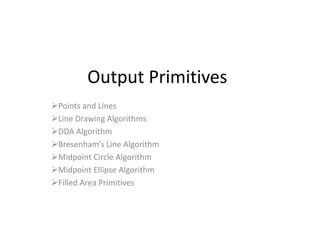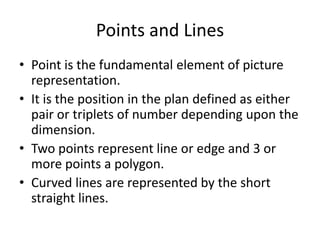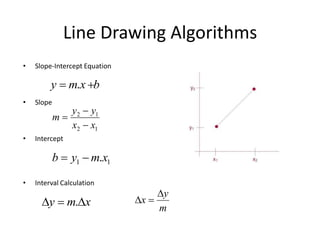Successfully reported this slideshow.

# Output primitives in Computer Graphics

49

Share×
1 of 80
1 of 80

# Output primitives in Computer Graphics

49

Share

This slide contain description about the line, circle and ellipse drawing algorithm in computer graphics. It also deals with the filled area primitive.

This slide contain description about the line, circle and ellipse drawing algorithm in computer graphics. It also deals with the filled area primitive.

## More Related Content

### Related Books

Free with a 14 day trial from Scribd

See all

### Related Audiobooks

Free with a 14 day trial from Scribd

See all

### Output primitives in Computer Graphics

1. 1. Output Primitives Points and Lines Line Drawing Algorithms DDA Algorithm Bresenham’s Line Algorithm Midpoint Circle Algorithm Midpoint Ellipse Algorithm Filled Area Primitives
2. 2. Points and Lines • Point is the fundamental element of picture representation. • It is the position in the plan defined as either pair or triplets of number depending upon the dimension. • Two points represent line or edge and 3 or more points a polygon. • Curved lines are represented by the short straight lines.
3. 3. Line Drawing Algorithms • Slope-Intercept Equation • Slope • Intercept • Interval Calculation bxmy  . 12 12 xx yy m    11 .xmyb  xmy  . m y x  
4. 4. Line Drawing Algorithm
5. 5. DDA Algorithm  Digital Differential Analyzer – Sample the line at unit intervals in one coordinate – Determine the corresponding integer values nearest the line path in another co-ordinate
6. 6. DDA Algorithm (left to right) • Slope • For |m|<1 (|Δy|< |Δx|) – Sample line at unit interval in x co-ordinate • For |m|>1 (|Δy|> |Δx|) – Sample line at unit interval in y co-ordinate x y xx yy m kk kk         1 1 myy kk 1 m xx kk 1 1  11   kk xxx 11   kk yyy
7. 7. DDA Algorithm (right to left) • Slope • For |m|<1 (|Δy|< |Δx|) – Sample line at unit interval in x co-ordinate • For |m|>1 (|Δy|> |Δx|) – Sample line at unit interval in y co-ordinate myy kk 1 m xx kk 1 1  11   kk xxx 11   kk yyy x y xx yy m kk kk         1 1
8. 8. DDA Algorithm 1. Input the two line endpoints and store the left endpoint in (x0,y0) 2. Plot first point (x0,y0) 3. Calculate constants Δx, Δy 4. If |Δx| > |Δy| steps = |Δx| else steps = |Δy| 5. Calculate XInc = |Δx| / steps and YInc = |Δy| / steps 6. At each xk along the line, starting at k=0, Plot the next pixel at (xk + XInc, yk + YInc) 7. Repeat step 6 steps times
9. 9. Pseudo Code Void lineDDA(int xa, int ya, int xb, int yb) { int dx = xb – xa, dy = yb – ya, steps, k; float xIncrement, yIncrement, x = xa, y = ya; if( abs (dx) > abs (dy) ) steps = abs (dx); else steps = abs (dy); xIncrement = dx / (float) steps; yIncrement = dy / (float) steps; setPixel (ROUND (x), ROUND (y)); for (k=0; k<steps; k++){ x += xIncrement; y += yIncrement; setPixel (ROUND(x), ROUND(y)); } }
10. 10. • Use DDA algorithm for rasterizing line (0,0) to (6,6). • Use DDA algorithm for rasterizing line (0,0) to (4,6).
11. 11. Bresenham’s Line Algorithm • Uses only incremental integer calculations • Which pixel to draw ? – (11,11) or (11,12) ? – (51,50) or (51,49) ? – Answered by Bresenham
12. 12. • For |m|<1 – Start from left end point (x0,y0) step to each successive column (x samples) and plot the pixel whose scan line y value is closest to the line path. – After (xk,yk) the choice could be (xk+1,yk) or (xk+1,yk+1)
13. 13. Then And Difference between separations bxmy k  )1( kyyd 1 kk ybxm  )1( yyd k  )1(2 bxmy kk  )1(1 122)1(221  byxmdd kk
14. 14. Defining decision parameter  Sign of pk is same as that of d1-d2 for Δx>0 (left to right sampling) For Recursive calculation, initially cyxxy kk  .2.2 Constant=2Δy + Δx(2b-1) Which is independent of pixel position cyxxyp kkk   111 .2.2 )(2)(2 111 kkkkkk yyxxxypp   c eliminated here )(22 11 kkkk yyxypp   because xk+1 = xk + 1 yk+1-yk = 0 if pk < 0 yk+1-yk = 1 if pk ≥ 0 xyp  20 Substitute b = y0 – m.x0 and m = Δy/Δx in  )( 21 ddxpk 
15. 15. Algorithm Steps (|m|<1) 1. Input the two line endpoints and store the left endpoint in (x0,y0) 2. Plot first point (x0,y0) 3. Calculate constants Δx, Δy, 2Δy and 2 Δy- 2Δx, and obtain p0 = 2Δy – Δx 4. At each xk along the line, starting at k=0, perform the following test: If pk<0, the next point plot is (xk+1,yk) and Pk+1 = pk + 2Δy Otherwise, the next point to plot is (xk + 1, yk+1) and Pk+1 = pk + 2Δy - 2Δx 5. Repeat step 4 Δx times
16. 16. What’s the advantage? • Answer: involves only the calculation of constants Δx, Δy, 2Δy and 2Δy- 2Δx once and integer addition and subtraction in each steps
17. 17. Example Endpoints (20,10) and (30,18) Slope m = 0.8 Δx = 10, Δy = 8 P0 = 2Δy - Δx = 6 2Δy = 16, 2Δy-2Δx = -4 Plot (x0,y0) = (20,10)
18. 18. • Use Bresenham’s algorithm for rasterizing the line (5,5) to (13,9)
19. 19. • Digitize a line with endpoints (15,18) and (10,15) using BLA.
20. 20. • Digitize a line with endpoints (15,15) and (10,18) using BLA.
21. 21. Algorithm Steps (|m|>1) 1. Input the two line endpoints and store the left endpoint in (x0,y0) 2. Plot first point (x0,y0) 3. Calculate constants Δx, Δy, 2Δx and 2 Δx- 2Δy, and obtain p0 = 2Δx – Δy 4. At each xk along the line, starting at k=0, perform the following test: If pk<0, the next point plot is (xk, yk+1) and Pk+1 = pk + 2Δx Otherwise, the next point to plot is (xk + 1, yk+1) and Pk+1 = pk + 2Δx - 2Δy 5. Repeat step 4 Δx times
22. 22. • Use BLA algorithm for rasterizing line (0,0) to (4,6).
23. 23. Circle-Generating Algorithms (Basic Foundations) • Circle Equation: • Points along circumference could be calculated by stepping along x- axis: 222 )()( ryyxx cc  22 )( xxryy cc 
24. 24. Problem (in above method) • Computational complexity • Spacing: – Non-uniform spacing of plotted pixels
25. 25. Adjustments (To fix problems) • Spacing problem (2 ways): – Interchange the role of x and y whenever the absolute value of the slope of the circle tangent > 1 – Use polar co-ordinate: • Equally spaced points are plotted along the circumference with fixed angular step size. • step size chosen for θ depends on the application and display device. • Computation Problem: – Use symmetry of circle; i.e calculate for one octant and use symmetry for others.   sin cos ryy rxx c c  
26. 26. Circle Symmetry
27. 27. Bresenham’s Algorithm Could Be Adapted ?? • Yes • How ? – Setting decision parameter for finding the closest pixel to the circumference • And what to do For Non-linear equation of circle ? – Comparison of squares of the pixel separation distance avoids square root calculations
28. 28. Midpoint Circle Algorithm • Circle function defined as: • Any point (x,y) satisfies following conditions         boundarycircletheoutsideisyxif boundarycircletheonisyxif boundarycircletheinsideisyxif yxfcircle ),(,0 ),(,0 ),(,0 ),( 222 ),( ryxyxfcircle 
29. 29. • Decision parameter is the circle function; evaluated as: 222 ) 2 1 ()1( ) 2 1 ,1( ryx yxfp kk kkcirclek   1)()()1(2 ) 2 1 (]1)1[( ) 2 1 ,1( 1 22 11 22 1 2 111       kkkkkkk kk kkcirclek yyyyxpp ryx yxfp
30. 30. yk+1 = yk if pk<0 yk+1 = yk-1 otherwise • Thus Pk+1 = Pk + 2xk+1+1 if pk<0 Pk+1 = Pk + 2xk+1+1-2yk+1 otherwise • Also incremental evaluation of 2xk+1 and 2yk+1 2xk+1 = 2xk + 2 2yk+1 = 2yk – 2 if pk >0 • At start position (x0,y0) = (0,r) 2x0 = 0 and 2y0 = 2r
31. 31. • Initial decision parameter • For r specified as an integer, round p0 to P0 = 1-r (because all increments are integers) r rr rfp circle    4 5 ) 2 1 (1 ) 2 1 ,1( 22 0
32. 32. Algorithm 1. Input radius r and circle center (xc, yc) and obtain the first point on the circumference of a circle centered on the origin as (x0,y0) = (0,r) 2. Calculate the initial value of the decision parameter as P0 = 5/4 – r 3. At each xk position, starting at k = 0, perform the following test: If pk < 0, the next point along the circle centered on (0,0) is (xk+1,yk) and Pk+1 = pk + 2xk+1 + 1 Otherwise, the next point along the circle is (xk+1,yK-1) and Pk+1 = pk + 2xk+1 + 1 -2yk+1 Where 2xk+1 = 2xk + 2 and 2yk+1 = 2yk-2 4. Determine the symmetry points in the other seven octants. 5. Move each calculated pixel position (x,y) onto the circular path centered on (xc,yc) and plot the co-ordinate values: x = x + xc, y = y+yc 6. Repeat steps 3 through 5 until x ≥ y
33. 33. • Given radius =10 use mid-point circle drawing algorithm to determine the pixel in the first quadrant. Solution: P0 = 1-r = -9 (x0 , y0)=(0,10) 2x0 =0 2y0 =20
34. 34. • Digitize the circle (x-2)^2+(y-3)^2=25 in first quadrant. Solution: P0=(1-r)= -4 (x0 , y0)=(0,5) 2x0 =0 2y0 =10
35. 35. Ellipse Generating Algorithms • Equation of ellipse: • F1(x1,y1), F2(x2,y2) • General Equation • Simplified Form • In polar co-ordinate constantyyxxyyxx  2 2 2 2 2 1 2 1 )()()()( constantdd  21 022  FEyDxCxyByAx 1 22                  y c x c r yy r xx   sin cos yc xc ryy rxx  
36. 36. • Ellipse function 222222 ),( yxyellipse rryrxryxf x         boundaryellipsetheoutsideisyxif boundaryellipsetheonisyxif boundaryellipsetheinsideisyxif yxfellipse ),(,0 ),(,0 ),(,0 ),(
37. 37. • From ellipse tangent slope: • At boundary region (slope = -1): • Start from (0,ry), take x samples to boundary between 1 and 2 • Switch to sample y from boundary between 1 and 2 (i.e whenever ) yr xr dx dy x y 2 2 2 2  yrxr xy 22 22  yrxr xy 22 22  Slope=-1
38. 38. • In the region 1 • For next sample • Thus increment 222222 ) 2 1 ()1( ) 2 1 ,1(1 yxkxky kkellipsek rryrxr yxfp                               22 1 222 1 222 1 222 111 2 1 2 1 )1(211 ) 2 1 (1)1( ) 2 1 ,1(1 kkxykykk yxkxky kkellipsek yyrrxrpp rryrxr yxfp         01,22 01,2 1 22 1 2 2 1 2 kkxyky kyky pifyrrxr pifrxr increment •For increment calculation; Initially: •Incrementally: Update x by adding 2ry 2 to first equation and update y by subtracting 2rx 2 to second equation yxx y rryr xr 22 2 22 02  
39. 39. • Initial value 222 0 22 2 22 0 4 1 1 2 1 2 1 ,11 xyxy yxyxy yellipse rrrrp rrrrr rfp                (0,ry)
40. 40. • In the region 2 • For next sample • Initially 222222 )1() 2 1 ( )1, 2 1 (2 yxkxky kkellipsek rryrxr yxfp                                      22 1 222 1 2222 2 1 2 111 2 1 2 1 )1(222 11 2 1 )1, 2 1 (2 kkyxkxkk yxkxky kkellipsek xxrryrpp rryrxr yxfp 222 0 2 2 0 2 0 000 )1( 2 1 2 1, 2 1 2 yxxy ellipse rryrxrp yxfp               For simplification calculation of p20 can be done by selecting pixel positions in counter clockwise order starting at (rx,0) and unit samples to positive y direction until the boundary between two regions
41. 41. Algorithm 1. Input rx,ry, and the ellipse center(xc,yc) and obtain the first point on an ellipse centered on the origin as (x0,y0) = (0,ry) 2. Calculate the initial value of the decision parameter in region 1 as 3. At each xk position in region 1, starting at k = 0, perform the following test: If p1k < 0, the next point along the ellipse centered on (0,0) is (xk+1,yk) and Otherwise, the next point along the ellipse is (xk+1,yk-1) and With and continue until 222 0 4 1 1 xyxy rrrrp  2 1 2 1 211 ykykk rxrpp   2 1 2 1 2 1 2211 ykxkykk ryrxrpp   22 1 222 1 2 222,222 xkxkxykyky ryryrrxrxr   yrxr xy 22 22 
42. 42. 4. Calculate the initial value of decision parameter in region 2 using the last point (x0,y0) calculated in region 1 as 5. At each yk position in region 2, starting at k = 0, perform the following test: If p2k>0, the next point along the ellipse centered on (0,0) is (xk,yk-1) and Otherwise the next point along the ellipse is (xk+1,yk-1) and Using the same incremental calculations for x and y as in region 1. 6. Determine the symmetry points in the other three quadrants. 7. Move each calculated pixel position (x,y) onto the elliptical path centered on (xc,yc) and plot the co-ordinate values: X = x + xc, y = y+ yc 8. Repeat the steps for region 1 until 222 0 2 2 0 2 0 )1( 2 1 2 yxxy rryrxrp        2 1 2 1 222 xkxkk ryrpp   2 1 2 1 2 1 2222 xkxkykk ryrxrpp   yrxr xy 22 22 
43. 43. • Digitize the ellipse with parameter rx =8 and ry =6 in first quadrant. Solution:
44. 44. Filled Area Primitives • Two basic approaches for area filling: – By determining overlap intervals for scan lines – Start from given interior position and filling outwards
45. 45. Scan Line Polygon Filled Algorithm • Intersection points of scan line with the polygon edge are calculated. • Points are sorted from left to right. • Corresponding frame buffer position between each intersection pair are set by specified color.
46. 46. • A scan line pass through vertex, intersect two polygon edges.
47. 47. • Scan line y’ – Intersect even number of edges – 2 pairs of intersection points correctly find the interior span • Scan line y – Intersect an odd number of edges(5) – Must count the vertex intersection as only one point
48. 48. How to distinguish these cases? • Scan line y – Intersecting edges are on the opposite side • Scan line y’ – Intersecting edges are on the same side. • By tracing around the boundary, – If the endpoint y values of two consecutive edges monotonically increases or decreases count middle vertex as single
49. 49. Implementation
50. 50. • In determining edge intersection, we can set up incremental set up calculation using fact that slope of edge is constant.
51. 51. Inside Outside test • Odd Even rule: – Odd edge means interior • Non zero winding number rule – Non zero winding number means interior
52. 52. Scan line fill for Curved Area • Require more works than polygon filling due to non-linear boundary. • We calculate the scan line intersection and fill all the interior points. • Symmetries between the quadrant can be used to reduce the calculation.
53. 53. Boundary fill algorithm • Fill the region starting from the interior point until the appropriate color boundary is reached. • Two ways: – 4 connected – 8 connected
54. 54. 4 connected
55. 55. 8 connected
56. 56. Using 4 connected flood fill algorithm